Courses

# Small Oscillations - Lagrangian and Hamiltonian Equations, Classical Mechanics, CSIR-NET Physical Sc Physics Notes | EduRev

## Physics for IIT JAM, UGC - NET, CSIR NET

Created by: Akhilesh Thakur

## Physics : Small Oscillations - Lagrangian and Hamiltonian Equations, Classical Mechanics, CSIR-NET Physical Sc Physics Notes | EduRev

The document Small Oscillations - Lagrangian and Hamiltonian Equations, Classical Mechanics, CSIR-NET Physical Sc Physics Notes | EduRev is a part of the Physics Course Physics for IIT JAM, UGC - NET, CSIR NET.
All you need of Physics at this link: Physics

Introduction

As an example of the use of the Lagrangian, we will examine the problem of small oscillations about a stable equilibrium point. The description of motion about a stable equilibrium is one of the most important problems in physics. This is true for both classical and quantum mechanics.
Although there are other means of solving this problem, the Lagrangian method results in equations of motion that emphasizes symmetries of the system.

1.1 Formulation of the Problem

We assume that the forces acting on the system are conservative in which the potential depends only on the spatial coordinates; it is independent of the time and velocity. In addition, the transformations de¯ning the generalized coordinates are assume to not depend on the time explicitly. Hence, the constraints are time independent. For the system to be in equilibrium, the generalized force must be zero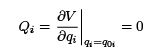(1)

where q0i is the equilibrium point. Furthermore, the equilibrium point is stable if the second derivative of potential is positive; the potential is concave up at the equilibrium point.
We will be interested in small displacement about the equilibrium. Therefore, we will write the generalized coordinates in the following form qi = q0i + ηi where ηi is the displacement from the equilibrium point. Assuming the displacements are small, the potential can be expanded about q0i as follows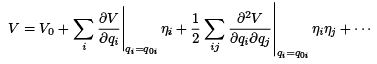(2)

The first term in the expansion is a constant, since the potential is only defined to a constant, we can ignore this term. The second term is zero, since we are expanding about the minimum. Therefore, the potential in this approximation is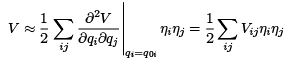(3)

where we assume that the displacements are small such that higher order terms are negligible.

The Vij in this equation are the equivalent spring constant for the one-dimensional problem. In addition, we note that the Vij are symmetric as can be seen from the definition.
The kinetic energy, as shown earlier, can be written as the sum of three terms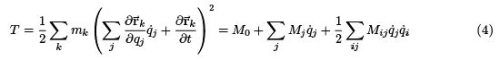where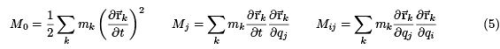Since we assume that the transformations are independent of time, only the Mij terms are nonzero; this is a natural system. In addition, since Mij in general is a function of the coordinates, we expand the Mij about the equilibrium point, since we are assuming small displacements about it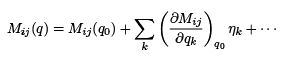(6)

We keep only the lowest order term in this expansion, since the kinetic energy is already quadratic in the coordinates (velocities). Thus, the kinetic energy becomes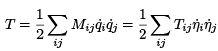(7)

where the relation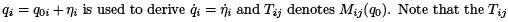terms are symmetric.
The Lagrangian for small oscillations about an equilibrium point is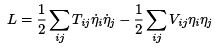(8)

where Tij describes the inertia of the system, and Vij the stiffiness1 . In Cartesian coordinates, and many other case, the kinetic energy term can be reduced to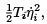the very familiar form of the kinetic energy. To calculate the equations of motion, we apply the Euler-Lagrange equations to the Lagrangian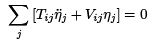(9)

Keep in mind that the Mij and Vij are independent of the coordinates and time, and they are symmetric.

The Eigenvalues and Eigenvectors

The solutions to the equations of motion are of the form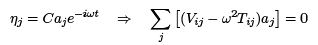(10)

where C is an overall scale factor, ai the relative amplitude of the ith term, and the second equation comes from substituting the solutions into the equations of motion. Notice that this is a matrix equation, representing matrix multiplication between and nxn matrix and an n-dimensional column vector. This set of equations can be solved for the allowed values of ! by setting the determinant of the coefficients of ai equal to zero.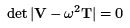(11)

where we have written the equation explicitly in matrix form.
Through the manipulations we have performed, we have arrived at an eigenvalue problem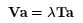(12)

where Va is shown to be a multiple of Ta. First we will show that the eigenvalues (λ) are real and positive, and the eigenvectors (a) are orthogonal. Start by taking the complex conjugate of Eq. 12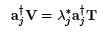(13)

where we have used the fact that V and T are real and symmetric, and in addition, j corresponds to the jth eigenvalue and eigenvector. Next, multiply Eq. 13 by ai from the right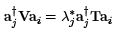(14)

For the ith eigenvalue and eigenvector, multiply Eq. 12 from the left by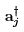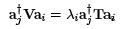(15)
Next, subtract the two equations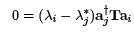If we assume that the λare distinct and we consider the case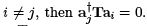This states that the amplitudes are orthogonal to each other with respect to T.
Let's now consider the case where i = j . First notice that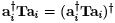implies that the matrix product is real. Next, write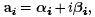where we have written ai in terms of its real and imaginary components. Then, we get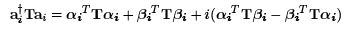(17)

Since the left hand side of this expression has already been shown to be real, the imaginary term is zero, thus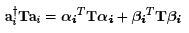(18)

Starting with Eq. 7, we see that in matrix notation the kinetic energy is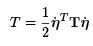(19)

Since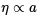and the phase of the amplitude is arbitrary, Eq. 18 represents twice the kinetic energy. For a real velocity, the kinetic energy must be positive. Therefore, the term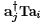must be positive (we already showed it was real), therefore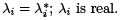Since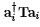is real, we can write ai as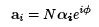(20)

where N is an overall normalization factor, and φ is a real phase. The two factors can be absorbed into C of Eq. 10 making the ai real.
Starting with the eigenvalue equation (Eq. 12) and multiplying from the left by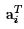we can solve for the individual eigenvalues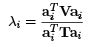(21)

The denominator is proportional to the kinetic energy, thus is must be positive. The numerator is the potential energy, which must be positive for a stable equilibrium. Therefore, λi must be positive and the frequencies are therefore real.
To remove the arbitrary normalization and phase from ai, we require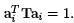Since we have an equation for each degree of freedom, we can combine this relation with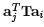= 0 and write a matrix A whose columns are the individual a. This leads to the relation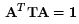(22)

which is a congruence transformation that diagonalizes the matrix T. We take this further by noting that Eq. 12 can be written as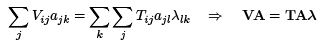(23)

where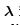is a diagonal matrix composed of the eigenvalues. If we multiply from the left by AT , we diagonalize V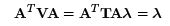(24)

Therefore, the problem of small oscillation is reduced to solving the following matrix problem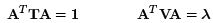(25)

That is, we must find a transformation that diagonalizes the matrix T. In this form, the Lagrangian is written as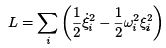(26)

1.3 Multiple Roots

Let's assume that two of the eigenvalues are equal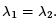. From the formalism given above, we expect that one of the eigenvectors will be orthogonal to the remaining eigenvectors, but there is no guarantee that they will be orthogonal to each other; recall that we wish to create the matrix A, which must be orthogonal in order to diagonalize the T and V matrices. To do this, we can write one of the eigenvector as the sum of the two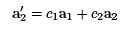(27)

and apply the orthogonality relation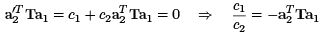(28)

This procedure can be generalized for n repeated roots (eigenvalues).

1.4 Example

Let's consider a triple pendulum as shown in Fig 1. We would like to determine the eigenfrequencies and eigenvectors (normal modes) of this oscillator for small oscillations.
We start by writing the Lagrangian in rectangular coordinates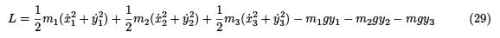where all the coordinates are measured from a coordinate system fixed to the pivot of the top pendulum as shown in the figure. Next we convert to a set of coordinates given by the angular displacement from the equilibrium point. The coordinates of the top pendulum are transformed as follows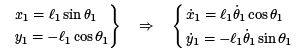(30)

The kinetic and potential energies for m1 are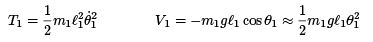(31)

where the approximation comes from expanding the potential in a Taylor series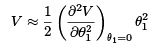(32)

Next, we consider the kinetic and potential energies associated with m2 . The transformation equations are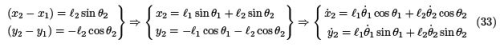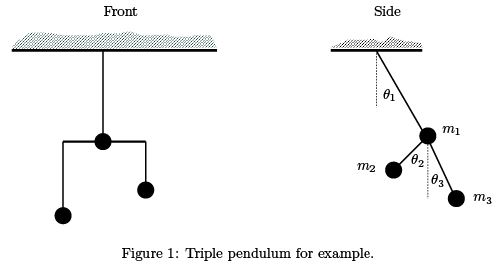Applying the transformations to the m2 terms, the kinetic and potential energies are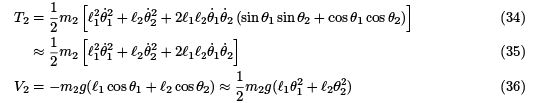The kinetic and potential energies associated with m3 are arrived at in a manner similar to m. These energies are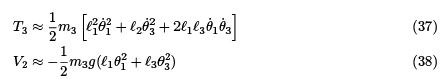Combining the kinetic and potential energy terms together, the Lagrangian becomes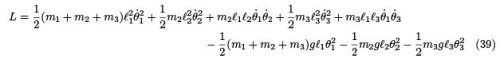To determine the eigenvalues, we write the kinetic and potential energies in matrix form. The mass matrix for the kinetic energy is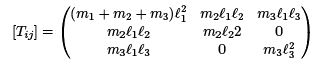(40)

and the stiffiness matrix is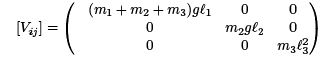(41)

Next, we solve the eigenvalue equation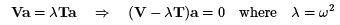(42)

The eigenvalues are given by setting the determinant of the coefficients of a equal to zero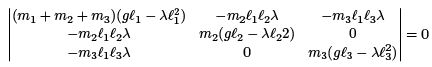(43)

Since the algebra becomes extremely complicated for arbitrary masses and lengths, we will take all the masses and length to be equal, in this case the determinant is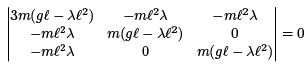(44)

and the eigenfrequencies are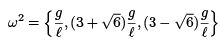(45)

Next, we calculate the motion of the normal modes. This is done by calculating the eigenvectors a for each eigenfrequency. We start with the the eigenfrequency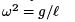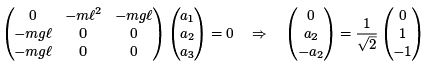(46)

We follow the same procedure for the two remaining eigenfrequencies and find the eigenvalues to be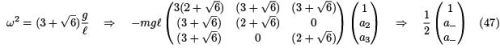where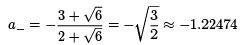(48)

Finally, repeat for the remaining eigenfrequency and we get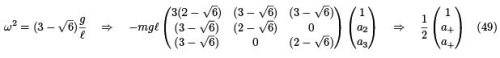where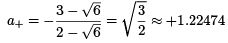(50)

What we have arrived at is the following description of the motion: The mode associated with the frequency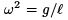has the upper mass stationary and the lower masses oscillating with the same amplitude, but π radians out of phase. The frequency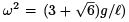corresponds to the two lower mass in phase and same amplitude, but π radians out of phase with the upper mass which has a smaller amplitude. The final mode corresponds to the two lower masses in phase with the upper mass, but the upper mass has a smaller amplitude.

Offer running on EduRev: Apply code STAYHOME200 to get INR 200 off on our premium plan EduRev Infinity!

159 docs

,

,

,

,

,

,

,

,

,

,

,

,

,

,

,

,

,

,

,

,

,

,

,

,

,

,

,

;